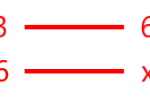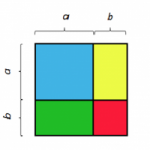Smartick is a fun way to learn math!# I want to learn about: Ratios & Proportional Relationships

Learn about ratios and proportional relationships with Smartick. Exercises, tutorials, problems, and teaching resources about ratios and proportional relationships. Here you will find posts about ratios and proportional relationships to make learning mathematics easier and more fun.

Feb11

## Rule of Three for Calculating PercentagesIn earlier posts, we have learned what a percent is and how to calculate it. Today we are going to use the rule of three to solve different types of problems related to percentages. Rule of three to calculate the percentage of a number For example, we want to calculate 30% of 360. 30% means […]

Jan07

## What Do Double and Half Mean?In today’s post, we are going to talk about the concepts double and half. First, I will explain what each term means and then we will see a strange situation with them. Double and half explain a type of relationship that exists between two numbers or quantities. Double of a quantity The double of a […]

Nov24

## Problems Involving the Inverse Rule of 3In this entry, we’re going to look at some problems involving the inverse rule of 3 so you can practice it at home. If you want to remind yourself of when you should apply the inverse rule of 3 and how to solve it, you can go to this previous blog entry: Inverse proportionality: The […]

Oct11

## Compound Rule of 3: When to Use It and Some ProblemsIn today’s post we’re going to take a look at the compound rule of 3, but first we need to remind ourselves about the simple rule of 3. I’ve selected some previous posts that you can look at if you need to: Rule of  three: Direct and Inverse Direct and Inverse Rule of three Problems […]

Aug25

## Inverse Proportionality: What Is It?Today, we’re going to learn about inverse proportionality between magnitudes. To start off, we need to remind ourselves that a magnitude is anything that can be measured. If this doesn’t ring a bell, look over our previous post where we talk about direct proportionality and explain the concept of magnitude: Direct Proportionality Lots of magnitudes […]

Aug02

## Proportional Relationship and Examples in Everyday LifeIn this post, we’re going to go a little further with proportional relationship. Let’s take a look at some different examples of ratio and proportion in everyday life. Before we begin, let’s review both of these concepts in the following link: Ratio and Proportion. As we’ve mentioned before, it’s all about two ways of relating quantities, […]

Jul28

## Notable Identities (Equalities or Notable Products)In today’s post we will look at how to calculate the square of the sum of two numbers a and b, (a + b) ². Notable Identities: For example: We built a square with sides of  a + b units length:   If we calculate the area of ​​this square (we know that the area […]

Jun23

## Proportional Numbers Problems. Ratio and ProportionIn today’s post we are going to work with proportions. Today, we’ll start by looking at problems with proportional numbers. In order to solve problems with proportional numbers, the first step is to know the concepts of ratio and proportion. Ratio A ratio is the quotient of two numbers or quantities that are being compared […]

Apr13

## Learn the Basic Concepts of the Rule of 3In this post, we’re going to take a look at when we can use the rule of 3 as a tool to solve a problem. The rule of 3 is one of the most applicable and most useful mathematical phenomena, above all due to its combination of simplicity and efficiency. We should take into account […]

Feb02

## Let’s Learn about Ratios with Singaporean Bar ModelsIn today’s post, we’re going to study the concept of ratios using the “Singapore Bar Model” methodology. First, we need to take into consideration that two quantities can be compared in different ways. By subtracting, by a fraction, and by multiplying…but today, we’re going to use ratios as a factor of comparison. To be able to […]